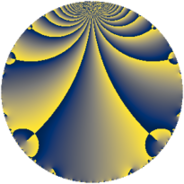# Properties

 Label 8470.2.a.caLevel $8470$ Weight $2$ Character orbit 8470.a Self dual yes Analytic conductor $67.633$ Analytic rank $1$ Dimension $2$ CM no Inner twists $1$

# Related objects

## Newspace parameters

 Level: $$N$$ $$=$$ $$8470 = 2 \cdot 5 \cdot 7 \cdot 11^{2}$$ Weight: $$k$$ $$=$$ $$2$$ Character orbit: $$[\chi]$$ $$=$$ 8470.a (trivial)

## Newform invariants

 Self dual: yes Analytic conductor: $$67.6332905120$$ Analytic rank: $$1$$ Dimension: $$2$$ Coefficient field: $$\Q(\sqrt{3})$$ Defining polynomial: $$x^{2} - 3$$ Coefficient ring: $$\Z[a_1, a_2, a_3]$$ Coefficient ring index: $$1$$ Twist minimal: yes Fricke sign: $$1$$ Sato-Tate group: $\mathrm{SU}(2)$

## $q$-expansion

Coefficients of the $$q$$-expansion are expressed in terms of $$\beta = \sqrt{3}$$. We also show the integral $$q$$-expansion of the trace form.

 $$f(q)$$ $$=$$ $$q + q^{2} + \beta q^{3} + q^{4} - q^{5} + \beta q^{6} + q^{7} + q^{8} +O(q^{10})$$ $$q + q^{2} + \beta q^{3} + q^{4} - q^{5} + \beta q^{6} + q^{7} + q^{8} - q^{10} + \beta q^{12} + \beta q^{13} + q^{14} -\beta q^{15} + q^{16} + ( -3 - \beta ) q^{17} -\beta q^{19} - q^{20} + \beta q^{21} + ( -1 - 2 \beta ) q^{23} + \beta q^{24} + q^{25} + \beta q^{26} -3 \beta q^{27} + q^{28} + ( -6 - 2 \beta ) q^{29} -\beta q^{30} + ( -5 - \beta ) q^{31} + q^{32} + ( -3 - \beta ) q^{34} - q^{35} + ( -4 + 2 \beta ) q^{37} -\beta q^{38} + 3 q^{39} - q^{40} + ( 5 - \beta ) q^{41} + \beta q^{42} + ( -1 - 5 \beta ) q^{43} + ( -1 - 2 \beta ) q^{46} + ( -1 + \beta ) q^{47} + \beta q^{48} + q^{49} + q^{50} + ( -3 - 3 \beta ) q^{51} + \beta q^{52} + ( -3 - \beta ) q^{53} -3 \beta q^{54} + q^{56} -3 q^{57} + ( -6 - 2 \beta ) q^{58} - q^{59} -\beta q^{60} -4 q^{61} + ( -5 - \beta ) q^{62} + q^{64} -\beta q^{65} + ( 9 - 3 \beta ) q^{67} + ( -3 - \beta ) q^{68} + ( -6 - \beta ) q^{69} - q^{70} + ( -4 - 4 \beta ) q^{71} + ( -1 + 5 \beta ) q^{73} + ( -4 + 2 \beta ) q^{74} + \beta q^{75} -\beta q^{76} + 3 q^{78} + ( 7 + 4 \beta ) q^{79} - q^{80} -9 q^{81} + ( 5 - \beta ) q^{82} + ( -5 + 4 \beta ) q^{83} + \beta q^{84} + ( 3 + \beta ) q^{85} + ( -1 - 5 \beta ) q^{86} + ( -6 - 6 \beta ) q^{87} + ( -3 + \beta ) q^{89} + \beta q^{91} + ( -1 - 2 \beta ) q^{92} + ( -3 - 5 \beta ) q^{93} + ( -1 + \beta ) q^{94} + \beta q^{95} + \beta q^{96} + ( -2 - 2 \beta ) q^{97} + q^{98} +O(q^{100})$$ $$\operatorname{Tr}(f)(q)$$ $$=$$ $$2q + 2q^{2} + 2q^{4} - 2q^{5} + 2q^{7} + 2q^{8} + O(q^{10})$$ $$2q + 2q^{2} + 2q^{4} - 2q^{5} + 2q^{7} + 2q^{8} - 2q^{10} + 2q^{14} + 2q^{16} - 6q^{17} - 2q^{20} - 2q^{23} + 2q^{25} + 2q^{28} - 12q^{29} - 10q^{31} + 2q^{32} - 6q^{34} - 2q^{35} - 8q^{37} + 6q^{39} - 2q^{40} + 10q^{41} - 2q^{43} - 2q^{46} - 2q^{47} + 2q^{49} + 2q^{50} - 6q^{51} - 6q^{53} + 2q^{56} - 6q^{57} - 12q^{58} - 2q^{59} - 8q^{61} - 10q^{62} + 2q^{64} + 18q^{67} - 6q^{68} - 12q^{69} - 2q^{70} - 8q^{71} - 2q^{73} - 8q^{74} + 6q^{78} + 14q^{79} - 2q^{80} - 18q^{81} + 10q^{82} - 10q^{83} + 6q^{85} - 2q^{86} - 12q^{87} - 6q^{89} - 2q^{92} - 6q^{93} - 2q^{94} - 4q^{97} + 2q^{98} + O(q^{100})$$

## Embeddings

For each embedding $$\iota_m$$ of the coefficient field, the values $$\iota_m(a_n)$$ are shown below.

For more information on an embedded modular form you can click on its label.

Label $$\iota_m(\nu)$$ $$a_{2}$$ $$a_{3}$$ $$a_{4}$$ $$a_{5}$$ $$a_{6}$$ $$a_{7}$$ $$a_{8}$$ $$a_{9}$$ $$a_{10}$$
1.1
 −1.73205 1.73205
1.00000 −1.73205 1.00000 −1.00000 −1.73205 1.00000 1.00000 0 −1.00000
1.2 1.00000 1.73205 1.00000 −1.00000 1.73205 1.00000 1.00000 0 −1.00000
 $$n$$: e.g. 2-40 or 990-1000 Significant digits: Format: Complex embeddings Normalized embeddings Satake parameters Satake angles

## Atkin-Lehner signs

$$p$$ Sign
$$2$$ $$-1$$
$$5$$ $$1$$
$$7$$ $$-1$$
$$11$$ $$1$$

## Inner twists

This newform does not admit any (nontrivial) inner twists.

## Twists

By twisting character orbit
Char Parity Ord Mult Type Twist Min Dim
1.a even 1 1 trivial 8470.2.a.ca yes 2
11.b odd 2 1 8470.2.a.bl 2

By twisted newform orbit
Twist Min Dim Char Parity Ord Mult Type
8470.2.a.bl 2 11.b odd 2 1
8470.2.a.ca yes 2 1.a even 1 1 trivial

## Hecke kernels

This newform subspace can be constructed as the intersection of the kernels of the following linear operators acting on $$S_{2}^{\mathrm{new}}(\Gamma_0(8470))$$:

 $$T_{3}^{2} - 3$$ $$T_{13}^{2} - 3$$ $$T_{17}^{2} + 6 T_{17} + 6$$ $$T_{19}^{2} - 3$$

## Hecke characteristic polynomials

$p$ $F_p(T)$
$2$ $$( -1 + T )^{2}$$
$3$ $$-3 + T^{2}$$
$5$ $$( 1 + T )^{2}$$
$7$ $$( -1 + T )^{2}$$
$11$ $$T^{2}$$
$13$ $$-3 + T^{2}$$
$17$ $$6 + 6 T + T^{2}$$
$19$ $$-3 + T^{2}$$
$23$ $$-11 + 2 T + T^{2}$$
$29$ $$24 + 12 T + T^{2}$$
$31$ $$22 + 10 T + T^{2}$$
$37$ $$4 + 8 T + T^{2}$$
$41$ $$22 - 10 T + T^{2}$$
$43$ $$-74 + 2 T + T^{2}$$
$47$ $$-2 + 2 T + T^{2}$$
$53$ $$6 + 6 T + T^{2}$$
$59$ $$( 1 + T )^{2}$$
$61$ $$( 4 + T )^{2}$$
$67$ $$54 - 18 T + T^{2}$$
$71$ $$-32 + 8 T + T^{2}$$
$73$ $$-74 + 2 T + T^{2}$$
$79$ $$1 - 14 T + T^{2}$$
$83$ $$-23 + 10 T + T^{2}$$
$89$ $$6 + 6 T + T^{2}$$
$97$ $$-8 + 4 T + T^{2}$$